# Lab: Tone Output Using An Arduino

## Introduction

This lab is an introduction to generating simple tones on an Arduino. In order to make the most of this lab, you should understand the basics of how to program digital input and output on an Arduino, and how to read a simple circuit diagram.

Video of this lab

## Making Sound Electronically

The following is adapted from SoundExamples.

Sound is created by vibrations in air. When those vibrations get fast enough, above about 20 times a second, you hear them as an audible pitch. The number of vibrations per second is called the frequency and frequency is measured in Hertz (Hz). So 20 times a second is 20Hz. Humans can hear pitches from about 20Hz to about 20,000Hz, or 20 kiloHertz (kHz).

What vibrations are we talking about? A speaker can vibrate. The paper cone of the speaker moves forward, then backward, then back to its resting place many times a second. The length of time it takes to move from rest through one back-and-forth motion, is called the period of the vibration. For example, if a speaker is vibrating at 20Hz, then it moves forward, backward, and back to rest 2in 1/20 of a second, or 0.05 seconds. 0.05 seconds is the period of the sound. Period and frequency are related inversely: frequency = 1/period.

A microcontroller makes sound by sending pulses of electrical energy from an output pin through a wire that’s connected to the paper cone of a speaker. That wire is wrapped in a coil, and mounted inside a magnet. The electrical energy generates a magnetic field, and that field is either attracted to the magnet or repelled by it, depending on which direction the electrical energy is flowing. The magnetic energy moves the coil, and since the coil is attached to the cone, the speaker moves.

So in summary: how do you make sound from an Arduino? Attach a speaker to an output pin and turn it on and off at a set frequency. You can write this code yourself, or you can use the `tone()` command.

## Pulsewidth Modulation vs. Frequency Modulation

When you use `analogWrite() `to create pulsewidth modulation (PWM) on an output pin, you’re also turning the pin on and off, so you might think, “why not use `analogWrite()` to make tones?” That command can change the on-off ratio of the output (also known as the duty cycle) but not the frequency. If you have a speaker connected to an output pin running `analogWrite()`, you’ll get a changing loudness, but a constant tone. To change the tone, you need to change the frequency. The `tone()` command does this for you.

## Prepare the breadboard

Connect power and ground on the breadboard to the microcontroller. On the Arduino module, use the 5V or 3.3V (depending on your model) and any of the ground connections. Figures 8 and 9 show connections for an Arduino Uno and a Nano, respectively.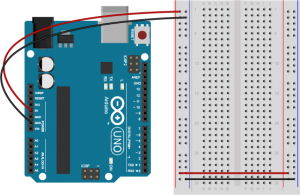Figure 8. Breadboard view of an Arduino Uno on the left connected to a solderless breadboard, right.

The Uno’s 5V output hole is connected to the red column of holes on the far left side of the breadboard (Figure 8). The Uno’s ground hole is connected to the blue column on the left of the board. The red and blue columns on the left of the breadboard are connected to the red and blue columns on the right side of the breadboard with red and black wires, respectively. These columns on the side of a breadboard are commonly called the buses. The red line is the voltage bus, and the black or blue line is the ground bus.

In Figure 9, the Nano is mounted at the top of the breadboard, straddling the center divide, with its USB connector facing up. The top pins of the Nano are in row 1 of the breadboard.

The Nano, like all Dual-Inline Package (DIP) modules, has its physical pins numbered in a U shape, from top left to bottom left, to bottom right to top right. The Nano’s 3.3V pin (physical pin 2) is connected to the left side red column of the breadboard. The Nano’s GND pin (physical pin 14) is connected to the left side black column. These columns on the side of a breadboard are commonly called the buses. The red line is the voltage bus, and the black or blue line is the ground bus. The blue columns (ground buses) are connected together at the bottom of the breadboard with a black wire. The red columns (voltage buses) are connected together at the bottom of the breadboard with a red wire.

Images made with Fritzing

## Connect the Sensors and the Speaker

Connect a variable resistor such as a force-sensing resistor or photosensor to analog pin 0 in a voltage divider circuit as shown below. The 8-ohm speaker connects to pin 8 of the Arduino. You can use any digital I/O pin if you don’t like 8. The other end of the speaker connects to ground. Figures 10 through 12 show the schematic drawing and the breadboard layouts for an Uno and a Nano, respectively.

Note: Although the circuit shown in Figures 10-12 has a 100-ohm resistor with the speaker, you can use a larger resistor. The larger the resistor, the quieter the speaker. A 220-ohm resistor works reasonably well if you don’t have a 100-ohm resistor.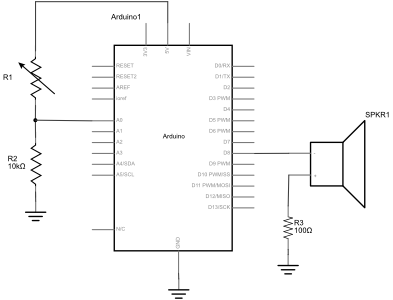Figure 10. Schematic view of an Arduino connected to a force sensing resistor (FSR), a 10-kilohm resistor, and a speaker. One leg of the FSR is connected to voltage. The other leg is connected simultaneously to the first leg of a 10-kilohm resistor and the Arduino’s analog input pin A0. The second leg of the 10-kilohm resistor is connected to ground. The red positive wire of the speaker is connected to digital pin 8 of the Arduino. The black ground wire of the speaker is connected to one leg of a 100 ohm resistor. The other leg of the resistor connects to ground.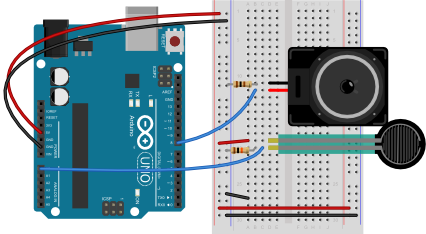Figure 11. Breadboard view of an Arduino connected to a force-sensing resistor, a fixed resistor, and a speaker. The Arduino’s voltage out and ground pins are connected to the voltage and ground buses of the breadboard as usual. The FSR is mounted in the left center section of the breadboard. One leg of the FSR is connected to 5 volts. The other leg is connected simultaneously to the first leg of a 10-kilohm resistor and the Arduino’s analog input pin A0. The second leg of the 10-kilohm resistor is connected to ground. The red positive wire of the speaker is connected to digital pin 8 of the Arduino. The black ground wire of the speaker is connected to one leg of a 100 ohm resistor. The other leg of the resistor connects to ground.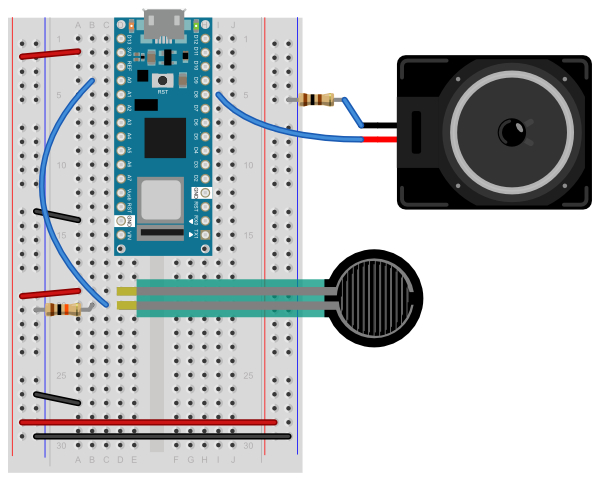Figure 12. Breadboard view of an Arduino Nano connected to two force sensing resistors (FSRs) and a speaker. The Nano’s 3.3 Volts (physical pin 2) and ground (physical pin 14) are connected to the voltage and ground buses of the breadboard as usual. The FSR is mounted below the Nano in the left center section of the breadboard. One leg of the FSR is connected to voltage. The other leg is connected simultaneously to the first leg of a 10-kilohm resistor and the Arduino’s analog input pin A0. The second leg of the 10-kilohm resistor is connected to ground. The red positive wire of the speaker is connected to digital pin 8 of the Arduino. The black ground wire of the speaker is connected to one leg of a 100 ohm resistor. The other leg of the resistor connects to ground.

## Check the sensor input range

Once you’ve got the circuit connected, check the range of the analog input and note the highest and lowest values you can reach.

```void setup() {
Serial.begin(9600);       // initialize serial communications
}

void loop() {
Serial.println(analogValue);      // print it
}
```

## Check that the Speaker Works

You can check that the speaker works by playing a single tone over and over. Here’s a short sketch to play middle A, 440Hz:

```void setup() {
// nothing to do here
}

void loop() {
// play the tone for 1 second:
tone(8, 440,1000);
// do nothing else for the one second you're playing:
delay(1000);
}
```

When you run this sketch, you should hear a tone of 44oHz, or middle A, continually. If you don’t, check to see that your speaker wiring is as shown above. If it’s too soft, try changing the 100 Ohm resistor to a smaller resistor.

## Play Tones

Write a sketch to read the analog input and map the result to a range from 100 to 1000. The example below assumes your analog input circuit ranges from 200 to 900, so adjuct for your actual values.  Store the result in a local variable called frequency. This will be the frequency you play on the speaker Then use the tone() command to set the frequency of the speaker on pin 8.

```void setup() {
// nothing to do here
}

void loop() {
// get a sensor reading:
// map the results from the sensor reading's range
// to the desired pitch range:
float frequency = map(sensorReading, 200, 900, 100, 1000);
// change the pitch, play for 10 ms:
tone(8, frequency, 10);
delay(10);
}
```

Once you’ve uploaded this, move your hands over the photocells, and listen to the frequency change. It will change from 100 Hz to 1000 HZ, because that’s what you set in the `map()` command. If you want to change the frequency range, change those two numbers. See if you can get it to play a little tune.

If you want to know how to generate a tone without the `tone()` command, here’s the basic algorithm to play one wavelength:

```void makeTone(float frequency) {
// set the period in microseconds:
int period = (1 / frequency) * 1000000;
// turn the speaker on:
digitalWrite(speakerPin, HIGH);
// delay half the period:
delayMicroseconds(period / 2);
// turn the speaker off:
digitalWrite(speakerPin, LOW);
// delay half the period:
delayMicroseconds(period / 2);
}
```

## Play it Loud

If you’d like to amplify the speaker, modify the speaker circuit by adding a transistor. Most any NPN transistor circuit or N-channel MOSFET will do. Figure 13 shows a speaker getting power from a transistor. This example uses a TIP120 transistor, but an NPN transistor or N-channel MOSFET should work. When using a transistor to amplify the power going to the speaker, you should definitely use a resistor to limit the current. A 100-ohm resistor is shown in this example.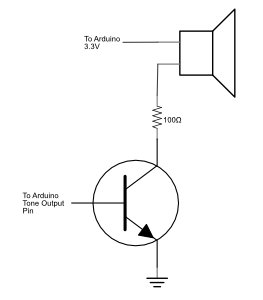Figure 13. Schematic view of a speaker connected to a transistor. The first leg of the speaker connects to the Arduino’s voltage supply (3.3V). The second leg of the speaker connects to a10-0-ohm resistor. The other side leg of the resistor connects to the collector pin of an NPN transistor (for a TIP120 transistor, this is the middle pin). The transistor’s base pin (the pin to the left when the transistor’s body is facing you) connects to the Arduino’s tone output pin (pin 8 in the examples above). The transistor’s collector pin (on the right when the transistor is facing you) connects to ground.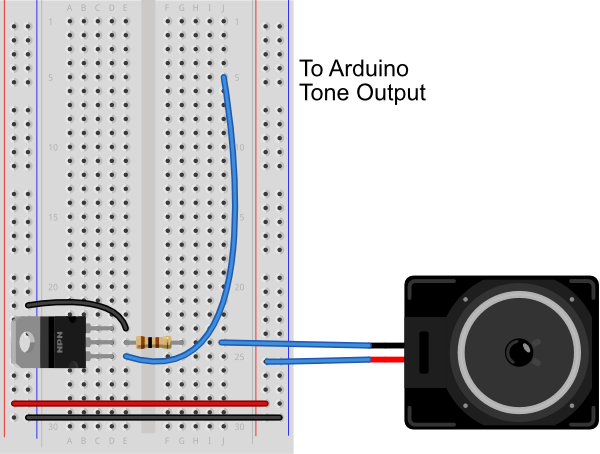Figure 14. Breadboard view of a speaker connected to a transistor. The speaker and transistor are connected exactly as described in the schematic view caption in Figure 13.

You can also connect the tone output of an Arduino to headphones. Using a standard 3.5mm audio jack, connect the center pin of the jack to ground through a 10-kilohm resistor. Then connect the two sides to each other and to your tone output pin. The 10-kilohm resistor here is important, because without it the audio signal will be too strong for your headphones, and could cause you ear damage. Figure 15 shows an audio jack connected to an Arduino Nano 33 IoT for the examples shown here.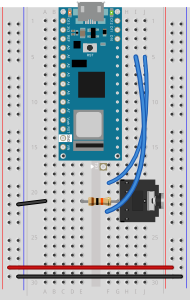Figure 15. Breadboard view of a 3.5mm Audio jack connected to an Arduino Nano 33 IoT. The center pin of the audio jack is connected to ground through a 10-kilohm resistor. The two sides are connected to the Nano 33’s tone output pin, which is pin 8 in this example.

## A more complex example

There’s an example in the Arduino IDE examples that can play a melody for you. Look in the File Menu under Examples -> Digital -> ToneMelody. You can find the code online at this link. In this example, you’ll see a second tab of text called `pitches.h`. This file includes constants that give you the pitches for a standard western scale.

You can add your own extra tabs using the new Tab menu to the right of the IDE window. Figure 16 shows the new Tab dropdown menu. Tabs make it easier to separate frequently copied code from custom code for a sketch, as you see in this example.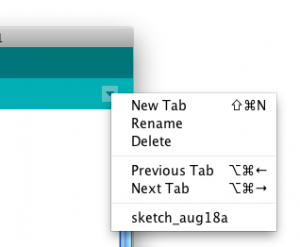Figure 16. A dropdown menu located in the upper right hand corner of the Arduino IDE showing the tab options. You can also type cmmand-shift-N to get a new tab.

You’ll notice that pitches.h lists a number of note names and numbers. Those numbers are the frequency of each note. For example:

```/*************************************************
* Public Constants
*************************************************/

#define NOTE_B0  31
#define NOTE_C1  33
#define NOTE_CS1 35
#define NOTE_D1  37
#define NOTE_DS1 39
#define NOTE_E1  41
#define NOTE_F1  44
#define NOTE_FS1 46
#define NOTE_G1  49
#define NOTE_GS1 52
#define NOTE_A1  55
#define NOTE_AS1 58
#define NOTE_B1  62
#define NOTE_C2  65
#define NOTE_CS2 69
#define NOTE_D2  73
```

This tells you that that first note in the list, B0 (which is the second lowest note on a piano keyboard, 4 octaves below middle A) has a frequency of 31 Hz. The values listed in `pitches.h` allow you to play notes from B0 to D#8.

For this sketch, you’ll play a simple melody. A melody consists of notes played in a sequence, and rests between the note. Each note and rest has its own particular duration. You’re going to play a seven note sequence, as shown in Figure 17 (in C Major):Figure 17. Two measures of a musical notation. The melody is “Shave and a haircut, two bits”

image from seventhstring.com

The sequence is: C4, G3, G3, G#3, G3, rest, B3, C4

the durations are:

quarter note, eighth note, eighth note, quarter note, quarter note, quarter rest, quarter note, quarter note.

The sketch starts with two global variables. Using pitches.h, make an array variable holding those notes. Make a second array to hold the note durations, marking quarter notes as 4 and eighth notes as 8.

```// notes in the melody:
int melody[] = {
NOTE_C4, NOTE_G3,NOTE_G3, NOTE_GS3, NOTE_G3,0, NOTE_B3, NOTE_C4};

// note durations: 4 = quarter note, 8 = eighth note, etc.:
int noteDurations[] = {
4, 8, 8, 4,4,4,4,4 };
```

How can you use the durations to play notes? Well, imagine that a quarter note is one quarter of a second, and eighth note is one eighth of a second, and so forth. In that case, the actual duration for each note is 1000 milliseconds divided by the value for it in the durations array. For example, the first note is 1000/4, the second is 1000/8, and so forth.

Now, you only want to play the song once, so everything will happen in the `setup()`. You’ll make a `for` loop in the setup to iterate over the seven notes. For each time through the loop, use `tone()` to play the next note in the array. Use the formula in the last paragraph to determine how long each note should play for. After you start the note, delay for as long as the note plays, plus 30 milliseconds or so, to separate each note.

```#include "pitches.h"

// notes in the melody:
int melody[] = {
NOTE_C4, NOTE_G3,NOTE_G3, NOTE_GS3, NOTE_G3,0, NOTE_B3, NOTE_C4};

// note durations: 4 = quarter note, 8 = eighth note, etc.:
int noteDurations[] = {4,8,8,4,4,4,4,4 };

void setup() {
// iterate over the notes of the melody:
for (int thisNote = 0; thisNote < 8; thisNote++) {
// to calculate the note duration, take one second
// divided by the note type.
//e.g. quarter note = 1000 / 4, eighth note = 1000/8, etc.
int noteDuration = 1000/noteDurations[thisNote];
tone(8, melody[thisNote],noteDuration);

//pause for the note's duration plus 30 ms:
delay(noteDuration +30);
}
}

void loop() {
// no need to repeat the melody.
}
```

That’s the whole tune!

## The Relation Between Pitches

For those interested in a little music theory, here’s where those frequency values in pitches.h came from. If you’re not interested in the details, feel free to skip to the next section.

You may have noticed in pitches.h that the value of B1 was twice that of B0, and that C1 is twice C0, and so forth.  In European classical music tradition, the most common tuning system in the last few hundred years has been the 12-tone equal temperament system. This system divides an octave into twelve pitches, in which the frequency interval between every pair of adjacent notes has the same ratio. The pitches are arranged on a logarithmic scale, and the ratio between pitches is equal to to the 12th root of 2, or 21/12, or approximately 1.05946. The whole tuning system is based off a reference frequency, 440 Hz, or middle A (a in the 4th octave, or A4). The step between each adjacent note is called a semitone. Wikipedia’s page on equal temperament gives a longer explanation if you want to know more. Musictheory.net is also a great source of information.

You can use this information to dynamically generate notes in this scale:

`frequency = 440 * 2^((noteValue - 69) / 12.0));`

This formula, taken from the MIDI tuning standard, works for a range of notes. Using this formula, note 27 is A0, the lowest note on a piano, and note 108 is C8, the highest note on a piano. 440 is the reference frequency, middle A, and 69 is the note number of middle A in MIDI. With this formula, you can cover a wide range of human hearing with note values 0 to 127. And you could generate note frequencies without needing `pitches.h`. This formula can be handy if you decide to dive into building MIDI devices later on as well. The Arduino API includes a function called the `pow()` function that raises a number to a power. So that formula, expressed as a line of Arduino programming code, would look like this:

`float frequency =  440 * pow(2, ((noteValue - 69) / 12.0));`

And the “shave and a haircut” tune from above, would be:

`int melody[] = {40, 35,35, 36, 35, 0, 39, 40};`

Expressed in note values like this, it would be simple to convert a musical sketch from playing using `tone()` to playing using a MIDI synthesizer.

Next, try making a musical instrument.

## A Musical Instrument

Playing a tune like you just doesn’t allow for much user interaction, so you might want to build more of a musical instrument.

Here’s an example of how to use the note constants to make a simple keyboard. Figures 18-20  show the circuit.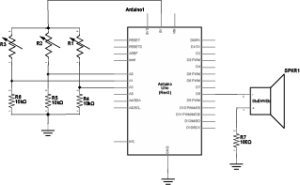Figure 18. Schematic view of an Arduino connected to three force sensing resistors and a speaker.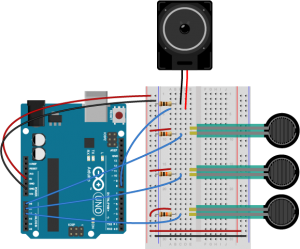Figure 19. Breadboard view of an Arduino connected to three force sensing resistors (FSR) and a speaker. Each of the three FSRs have one of their respective legs connected to +5 volts. Each of the other legs connect to one leg of a 10-kilohm resistor and simultaneously connect to one of the Arduino’s analog input pins. In this case the pin connections are A0, A1, and A2. Each of the respective 10-kilohm resistors then connect to ground. The red positive wire of the speaker is connected to digital pin 8 of the Arduino. The black ground wire of the speaker is connected to one leg of a 100 ohm resistor. The other leg of the 100 ohm resistor then connects to ground.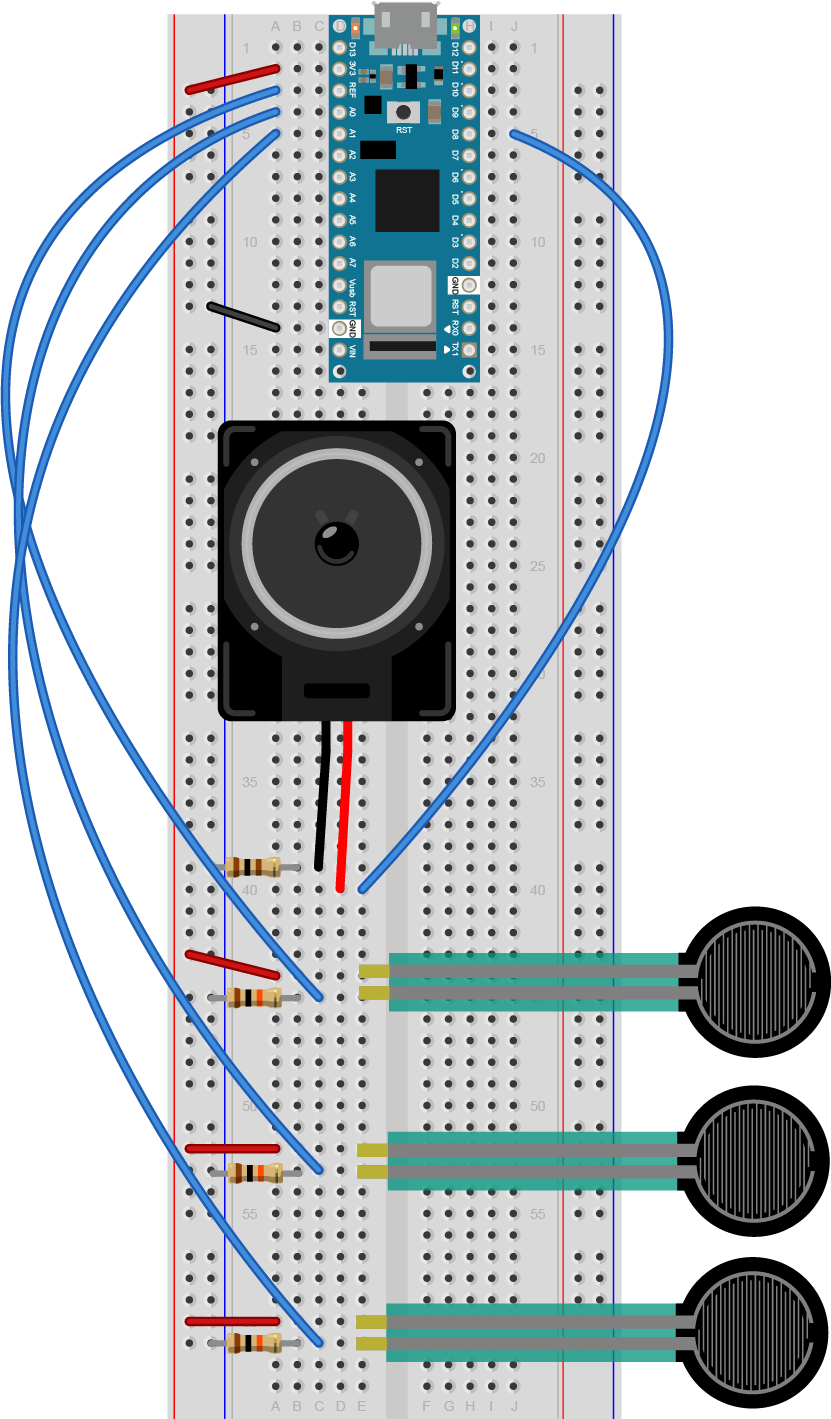Figure 20. Breadboard view of an Arduino Nano connected to three force sensing resistors (FSR) and a speaker. Each of the three FSRs have one of their respective legs connected to the voltage bus of the breadboard. Each of the other legs connect to one leg of a 10-kilohm resistor and simultaneously connect to one of the Arduino’s analog input pins. In this case the pin connections are A0, A1, and A2. Each of the respective 10-kilohm resistors then connect to ground. The red positive wire of the speaker is connected to digital pin 8 of the Arduino. The black ground wire of the speaker is connected to one leg of a 100 ohm resistor. The other leg of the 100 ohm resistor then connects to ground.

## Program it

Make a sketch that plays a note on each sensor when the sensor is above a given threshold.

Start with a few constants: one for the threshold, one for the speaker pin number, and one for the duration of each tone. To make this instrument work, you need to know what note corresponds to each sensor. Include pitches.h as you did above, then make an array that contains three notes, A4, B4, and C3 as well. Set all of this up at the beginning of your sketch, before `setup()`.

```#include "pitches.h"

const int threshold = 10;      // minimum reading of the sensors that generates a note
const int speakerPin = 8;      // pin number for the speaker
const int noteDuration = 20;   // play notes for 20 ms

// notes to play, corresponding to the 3 sensors:
int notes[] = {
NOTE_A4, NOTE_B4,NOTE_C3 };
```

You don’t need anything in your `setup()`, but in your loop, you need a for loop that iterates over the first three analog inputs, 0, 1, and 2. For each one, read the sensor, and if it’s above a threshold, play the corresponding note in the notes array for the note duration.

```#include "pitches.h"

const int threshold = 10;      // minimum reading of the sensors that generates a note
const int speakerPin = 8;      // pin number for the speaker
const int noteDuration = 20;   // play notes for 20 ms

// notes to play, corresponding to the 3 sensors:
int notes[] = {
NOTE_A4, NOTE_B4,NOTE_C3 };

void setup() {

}

void loop() {
for (int thisSensor = 0; thisSensor < 3; thisSensor++) {
// get a sensor reading:

// if the sensor is pressed hard enough:
if (sensorReading > threshold) {
// play the note corresponding to this sensor:
tone(speakerPin, notes[thisSensor], noteDuration);
}
}
}
```

When you upload this sketch, you should have a three-key keyboard.

## Get Creative

Now that you’ve got the basics, think about making an instrument for one of your projects. For more background on musical structure, see these notes on tone and generating a melody.